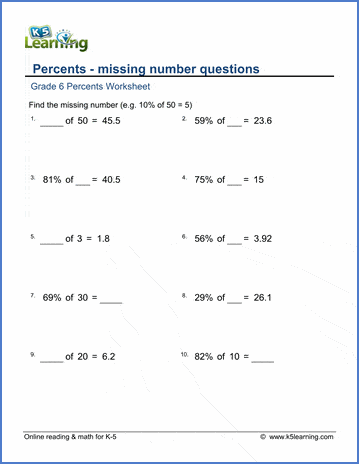# Percents Worksheets

## Math worksheets: Percents

These worksheets provide practice in common calculations involving percents, including changing decimals to and from percents, finding percentages of numbers and fining how many percent a number is of another number.

## Percents and fractions

Fractions to percents 7 / 10 = ___%
Percents to fractions 15% = 15/100  = 3/20

## Percents and decimals

Decimals to/from percents 0.65 = ___%
Decimals to/from percents (over 100%) 1.54 = ___ %

Fractions, decimals and percents 3/100 = 0.03 = 3%

## Percents of numbers

Percentages of numbers 70% of 80 =
Percentages (over 100%) of numbers 500% of 40 =
Percentages of numbers, harder 30% of 44 =
Percentages of numbers, harder-2 93% of 21 =

Percentages of decimal numbers 60% of 9.59 =

How many percent of X is Y 60 = ___% of 65
Percentages of number, missing number 70% of ___ = 56